# 0 引言

$$U(t)=A(t)-L(t),t>0$$

$$U(t)=u+ct-S(t);t\ge 0,u\ge 0,c>0$$

$$S(t)=\begin{cases}\\\ X_{1}+X_{2}+\cdots+X_{N_{(t)}} & ,N_{(t)}>0\\<br /> 0 & ,N_{(t)}=0\\\ \end{cases}$$

$N_{(t)}$表示$[0,t]$内的总理赔次数，$X_i$表示[0,t]$内第$i$次理赔的金额。 根据这个古典盈余过程模型可以引出破产模型，在这个盈余过程模型中，一方面有连续不断的保费收入并以速度c进行积累，另一方面则是不断会有理赔需要支付，因此这是一个不断跳跃变化的过程。从保险人的角度来看，当然希望$ct-S(t)$恒大于0，否则就有可能出现$U(t)<0$的情况，这种情况可以定义为理论意义上的破产，以示与实际中的破产相区分，本文中后面出现的“破产”在没有特殊说明的情况下都是指这种理论情况。从研究保险人破产角度出发，可以把这个盈余过程模型看做是一个特殊的破产模型。 # 1 第一个推广的破产模型 在以上经典模型中，假设了保费收入速度是均匀的，而在实际中，在控制保费c的条件下，保单到达的时刻应该是一个离散的随机过程。根据现实经验，考虑一段很短的时间间隔中，认为保单到达的概率较小，而时间间隔数量可以非常之多且不清楚具体是多少，在概率论中一般用泊松分布来刻画这种概率分布，所以初步认为一段时间内保单到达的数量服从泊松分布。 同样地，由于理赔发生的概率远比保单发生的概率低，因此可以认为理赔发生的次数服从另一个独立的泊松分布。选取泊松分布来刻画这两个时间间隔的另一个原因是泊松分布具有一些优良的数学性质，便于分析和计算。根据泊松分布的性质，保单到达和理赔到达的时刻是两个独立的泊松过程。 另外，一般一款保险产品，它的保费往往是固定的，所以用固定的c来表示符合现实情况，而理赔金额往往根据发生事故的严重程度而定，可以认为每次理赔的金额服从一个独立的取值为非负的分布，根据经验，这个分布大致的要求是较高的概率对应较小的理赔额，较低的概率对应较大的理赔额，在常用的概率分布中，指数分布较好地满足这个特性，本文初步选用指数分布来刻画每次理赔额。 因此，第一个推广的破产模型可以表示为： $$U(t)=u+cM(t)-\sum_{i=1}^{N(t)}X_i;t\ge 0,u\ge 0,c>0$$ 其中保单到达时刻$M(t)$服从参数为$\lambda_1$的泊松过程，理赔发生时刻$N(t)$服从参数为$\lambda_2$的泊松过程，每次支付的保险费$X_i$服从参数为$v$的相互独立的指数分布。 在这个模型中，保险人期望$cM(t)-\sum_{i=1}^{N(t)}X_i$能恒大于0，因此至少$E(cM(t)-\sum_{i=1}^{N(t)}X_i)>0$$c\lambda_1>\lambda_2/v$，另外从经验来看，保险事故发生的概率一般不高，一次理赔的的金额应该远大于收到的保费，所以保单到达的速率应该远比理赔发生的速率大，否则这种产品就没有经营价值，保险人也将面临破产，所以$\lambda_1\gg \lambda_2$ 考虑以下一个具体的破产模型案例： 某一款保险产品，假设保单到达的速率为$\lambda_1=10$张/天，理赔发生的速率为$\lambda_2=1$次/天。假设每张保单价格c=120，理赔额服从参数为$v=1/1000$（以$c\lambda_1=1.2\lambda_2/v$设定）的指数分布。设定初始$u=3000$时，计算到第1000天为止发生破产的概率。 本文用R语言模拟了10000次，用时1625秒，大概不到半个小时的时间，时间还能接受。最终结果10000次中破产5293次，破产率大概53%。输出各阶段破产时刻频数和频率结果如下：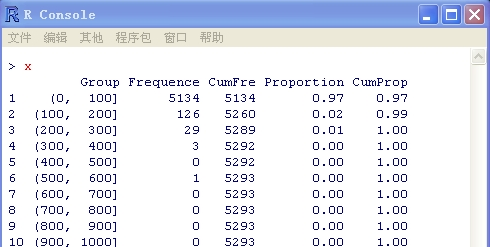直方图为：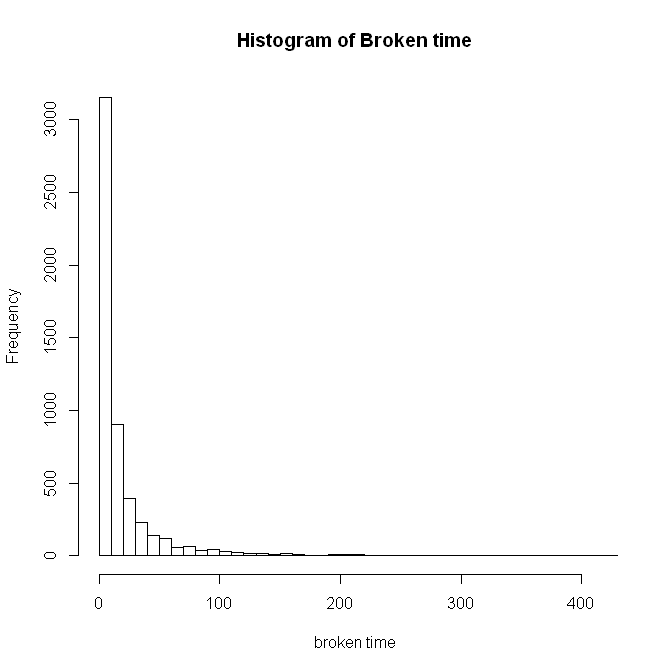由直方图可以非常明显地看出绝大部分破产时刻都在前100天，或者说从0开始的一小段时间内，在这之后的很长时间里，破产的频率急剧减少，可以认为破产的概率同样非常小。这对保险人来说，说明3000的初始盈余不够用，保险人需要准备更多准备金，才能抵抗初期的破产风险。 # 2 第二个继续推广的破产模型 在上一个破产模型中考虑了一款保险产品和对应理赔的问题，但现实中保险人往往同时经营着多种不同的保险产品，同样会有多种对应的理赔问题。基于这个想法，考虑将上一个模型进一步推广，就得到了以下模型： $$U(t) = u + \sum^{N(t)}_{i = 1}c_iM_i(t) – \sum^{N_i(t)}_{j = 1}\sum^{n}_{i = 1}X_{ij}, \quad t \geq 0; u \geq 0; c_i > 0$$ 将模型展开，可以表示为： $$U(t) = u + \sum^{N(t)}_{i = 1}c_iM_i(t) – (\sum^{N_1(t)}_{j = 1}X_{1j} + \sum^{N_2(t)}_{j = 1}X_{2j} + \cdots + \sum^{N_n(t)}_{j = 1}X_{nj})$$ 这个模型考虑有n款不同的保险产品，保费分别用$c_i$表示，第i款产品的保单到达时刻为$M_i(t)$。这样需要对应n款产品的理赔，假设第i款产品的理赔到达时刻为$N_i(t)$，对应的理赔额为$X_{ij}$，表示第i款产品第j次的理赔额。根据上一个模型的情况，这里假设$M_i(t)$是服从参数为$\alpha_i$的泊松过程，$N_i(t)$是服从参数为$\beta_i$的泊松过程，$X_{ij}$是服从参数为$v_i$的指数分布。同样地，根据每个保单的对应情况，要求满足$c_i \alpha_i > \beta_i / v_i$$\alpha_i > \beta_i$ 考虑以下一个具体的破产模型案例： 某保险人同时经营了三款不同的保险产品，假设保单到达的速率为$\alpha_i = 10$每一单位时间（简化起见，不考虑实际单位，改用形式的一单位时间），$\alpha_i = 20$$\alpha_i = 30$。理赔发生的速率为$\beta_i = 1$$\beta_i = 2$$\beta_i = 3$$X_{1j}$服从参数$v_1 = 1/1000$的指数分布，假定$v_2 = 1/1500$$v_3 = 1/2000$。以（20%预期收益率）的标准计算$c_i$的值。$c_1 = 120$$c_2 = 180$$c_3 = 240$ 鉴于上个模型的模拟经验，设置一个较大的初始盈余，初步设定$u = 10\$万。但是模拟结果发现破产率百分之一百，继续提高初始盈余，发现在把准备金提高到100万的情况下结果仍然全部破产，这充分说明破产概率主要不是由初始盈余决定的。在事故发生的概率，即理赔到达强度参数不可控的情况下，保险人可调整的参数剩下保费和理赔额服从指数分布的参数。

1000次模拟花时845秒，模拟结果破产概率27.7%，破产时刻的分布如下：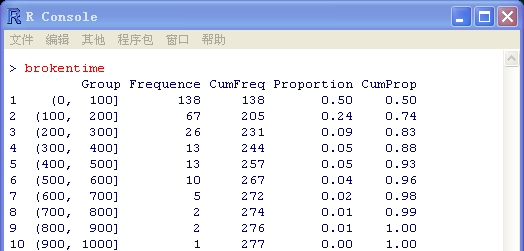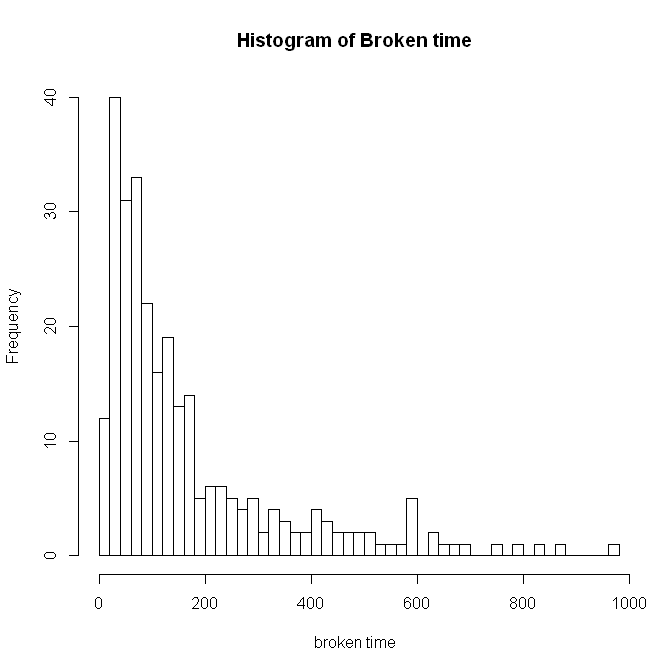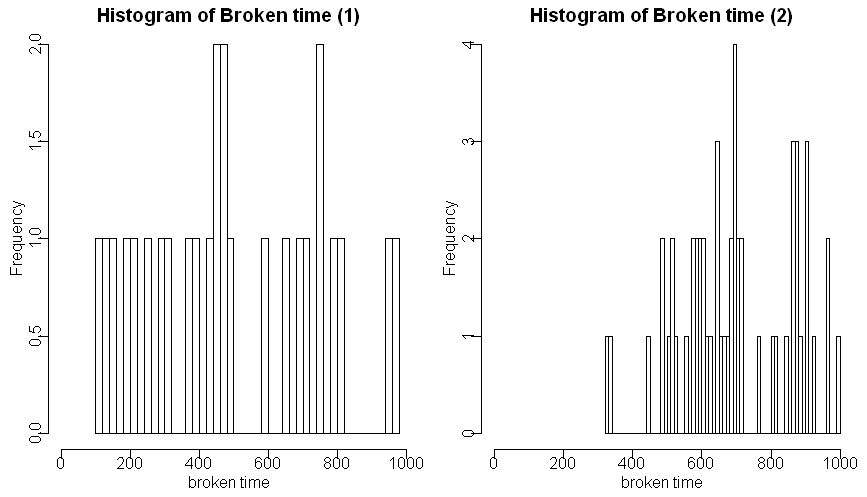# 参考文献

赵晓凡,牛明飞.广义多元风险模型破产时刻的随机模拟分析[J].中国科技论文,2008.

孙立娟,顾岚.保险公司破产概率的估计及随机模拟[J].系统工程理论与实践,2000.

陈新美.二元广义复合双Poisson风险模型下的破产概率[J].湘潭大学自然科学学报,2006.

廖基定.经典风险模型破产概率及其局部渐近解[J].应用数学学报,2009.

蒋云贵,柳思维.基于回归方程的流通企业破产风险模型[J].系统工程,2010.

吴岚,王燕.风险理论[M].中国时政经济出版社,2006.

肖枝洪,朱强.统计模拟及其R实现[M].武汉大学出版社,2010.

 [美]sheldon M.Ross.统计模拟(中文版·第4版)[M].人民邮电出版社,2007.

 [美]詹姆斯·埃文斯,戴维·奥尔森.模拟与风险分析[M].上海人民出版社,2001.

 [美] sheldon M.Ross.随机过程[M].中国统计出版社,1997.

何书元.随机过程[M].北京大学出版社,2008.

李皞.用R也能做精算—actuar包学习笔记